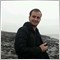# Subject: Does a logic operator works this way?1002

simple question:

normally you write:

```double statement = OrderTakeProfit();
double tp1 = 1.001;
double tp2 = 1.002;
double tp3 = 1.003;
double tp4 = 1.004;

bool TP_Exist = false;
if(statement == tp1)TP_Exist = true;
if(statement == tp1)TP_Exist = true;
if(statement == tp1)TP_Exist = true;
if(statement == tp1)TP_Exist = true;

return(TP_Exist);```

It is a long line of code to check if there is an Takeprofit on a trade. Will it also work with this?

```double statement = OrderTakeProfit();
double tp1 = 1.001;
double tp2 = 1.002;
double tp3 = 1.003;
double tp4 = 1.004;

bool TP_Exist = false;
if(statement == (tp1 || tp2 || tp3 || tp4)TP_Exist = true;

return(TP_Exist);```

To compare 1 statement if, with OR, OR,OR,OR in smallest code as possible20228

1. Willem Huisman: normally you write:
`if(statement == tp1)TP_Exist = true;`
No you don't. Doubles are rarely equal. Understand the links in:
The == operand. - MQL4 and MetaTrader 4 - MQL4 programming forum

2. Willem Huisman: It is a long line of code to check if there is an Takeprofit on a trade. Will it also work with this?
`if(statement == (tp1 || tp2 || tp3 || tp4)TP_Exist = true;`
non-zero is true. Therefor tp1||tp2||tp3||tp4 is true||true||true||true which is true. True is one. Therefor you are testing statement == 1.0000001002

whroeder1:
1. No you don't. Doubles are rarely equal. Understand the links in:
The == operand. - MQL4 and MetaTrader 4 - MQL4 programming forum

2. non-zero is true is one. Therefor you are testing TP = 1.000000

Basicly the idea was to manage the trades. I use the TP function of a trade to determine where they belong. For example when close signal is given, all trades with Symbol, type, must be selected to close. Cant do it with magic number since they are changing from categorie